Peleton

Cycling race was run at an average speed of 45 km/h. One cyclist lost with defect 8 minutes. How long and how far he must go at speed 51 km/h to catch peleton again?

Result

t =  60 min
s =  51 km

Solution:Leave us a comment of this math problem and its solution (i.e. if it is still somewhat unclear...):Be the first to comment!To solve this verbal math problem are needed these knowledge from mathematics:

Do you want to convert length units?

Next similar math problems:

1. Pedestrian and the cyclist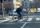Pedestrian and cyclist went against each from the two cities that are distant 40 km. Pedestrian started 30 minutes before the cyclist and walk an average speed of 5 km/h. What speed went cyclist when met after 120 minutes from the start of the pedestrian?
2. Bob traveled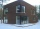Bob traveled 20 meters to Dave's house. He arrived in 3 minutes. What was his average speed for the trip.
3. Truck and car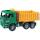At 6h45min the truck started at a speed of 36 km/h. At 7h30m behind it a passenger car. Find the average speed of passenger car if it will catch truck 72 km away from the factory.
4. Two cars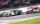Car A1 goes at average speed of 106 km/h and the second car A2 goes at 103 km/h. How many second will it take car A1 to circulate car A2? Assume that both cars are 5 meters long and the safety gap between cars is 1.5 meters.
5. Train expres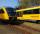At 8.00 strarted from Bratislava to Poprad express Tatry. Cities are distant 340 kilometers. At the same time started from Poprad express Danube to Bratislava. Express Tatry's average speed is 80 km/h, Danube 90 km/h. At what distance from Poprad trains w
6. Cyclist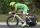A cyclist is moving at 35 km/h and follow pedestrian who is walking at speed 4 km/h. Walker has an advantage 19 km. How long take cyclist to catch up with him?
7. Double-track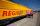On double-track express met with a freight train. Express travel at speed 72 km/h, a freight train at speed 36 km/h. After what time period will be from 9 km apart?
8. Round-tripA woman works at a law firm in city A which is about 50 miles from city B. She must go to the law library in city B to get a document. Find how long it takes her to drive​ round-trip if she averages 40 mph.
9. Skier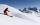At this point, the first skier lead 20 km before the second skier and travels at a constant speed 19 km/h. The second skier rides at 24 km/h. How long take him to catch up the first?
10. Subway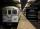Subway train went between two stations that gradually accelerated for 26 seconds and reached a speed of 72 km/h. At this rate, went 56 seconds. Then 16 seconds slowed to a stop. What was the distance between the stations?
11. Passenger car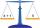Passenger car travels 1 km for 2.3 minutes, a truck during a 50 seconds long. How much is the speed of the truck less?
12. Runner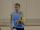Peter ran a speed of 12.4 km / h. After 45 minutes running he had a hiatus. The track is 31 km long. How many kilometers he still to run if he still running at the same speed?
13. WavelengthCalculate the wavelength of the tone frequency 11 kHz if the sound travels at speeds of 343 m/s.
14. Cars 6At 9:00 am two cars started from the same town and traveled at a rate of 35 miles per hour and the other car traveled at a rate of 40 miles per hour. After how many hours will the cars be 30 miles apart?
15. StormSo far, a storm has traveled 35 miles in 1/2 hour in direction straight to observer. If it is currently 5:00 p. M. And the storm is 105 miles away from you, at what time will the storm reach you? Explain how you solved the problem.
16. Theorem proveWe want to prove the sentence: If the natural number n is divisible by six, then n is divisible by three. From what assumption we started?
17. Freight and passenger car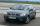The truck starts at 8 pm at 30 km/h. Passenger car starts at 8 pm at 40 km/h. Passenger arrives in the destination city 1 hour and 45 min earlier. What is the distance between the city of departure and destination city?